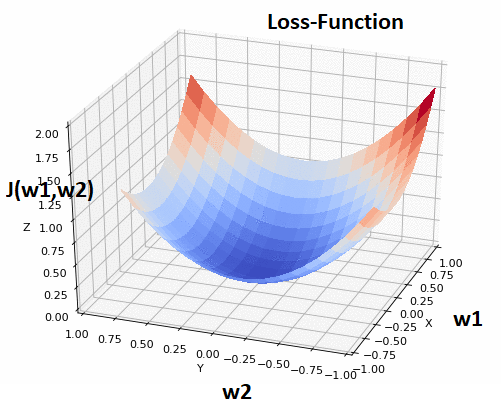﻿ Applied Roots

# In the end, Machine-Learning is all about Optimization: ft. Gradient DescentWe all know Machine-Learning is all about solving complex optimization problems, it can be a simple linear-regression with few weights or a Deep Neural-Network with millions of weights to train. Here training the weights essentially means minimizing the Loss-function(through which we measure our model’s performance)by finding the optimal weights, that represents a mathematical solution to the optimization problem using Gradient Descent.